# GSoC Blog | Activeloop | Week 7

lowlypalace
Published: 09/11/2022

This week, I had a quick sync with the mentors as it seemed like I couldn't derive systematic results from running my previous experiments.

I liked the idea to introduce some noise to some dataset that has a low rate (e.g. less than 1-3% of misclassified labels to compare baseline with cleanlab. I used Fashion MNIST and introduced some random corruption to the training set by flipping the labels.

In one of my experiments, I set the maximum noise to 50% and gradually introduced 5% the noise at each step, comparing the performance of baseline and cleanlab in parallel. This time, instead of relabelling, I decided to prune the samples with low confidence scores. Here’s a quick example: if I have 60,000 samples in the dataset, at 10% of the noise, I’d randomly flip 6,000 labels. The baseline would then be trained with all samples (60,000), but the cleanlab would be trained only on the labels that weren’t classified as erroneous by cleanlab . For example, If cleanlab found that 5,000 are labeled incorrectly, then I would only use 55,000 images for training.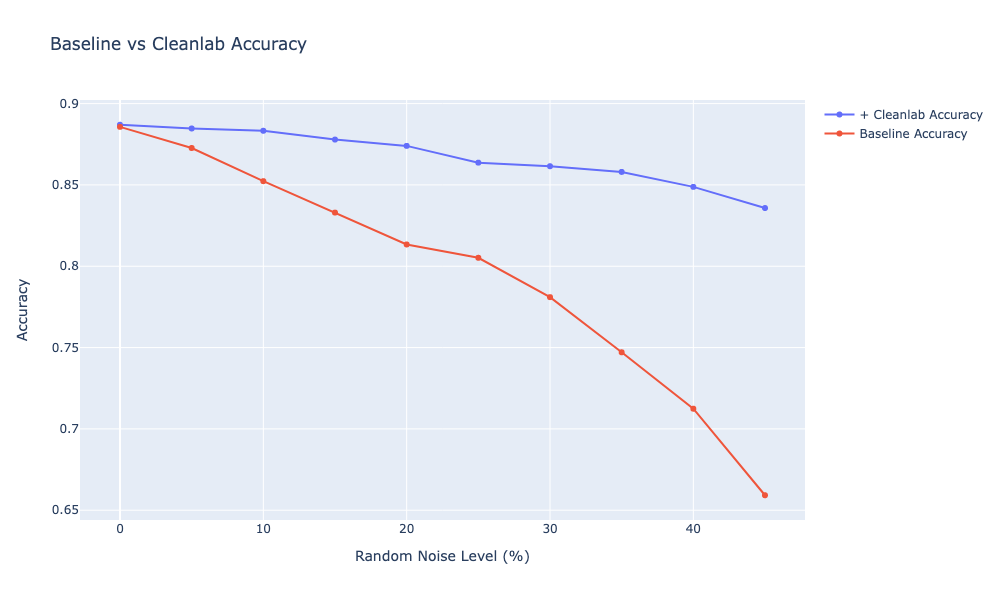It seems that pruning the samples with lower confidence works well, as cleanlab seems to remove the labels that were introduced with each noise level. We can also see that the accuracy stays around 80% with cleanlab, while with the random noise (e.g. without removing any samples) it drops linearly. On average, I can also see that cleanlab  on average prunes more labels than I initially introduced in the data. The mean of additional samples that cleanlab discards is ≈4500 samples across all noise levels. Since I don’t know the true noise in the original dataset, it’s hard to say whether cleanlab is doing a good job on removing these, but I would argue that cleanlab seems to be overestimating. However, it seems to systematically pick up the newly introduced noisy labels and identify them as erroneous.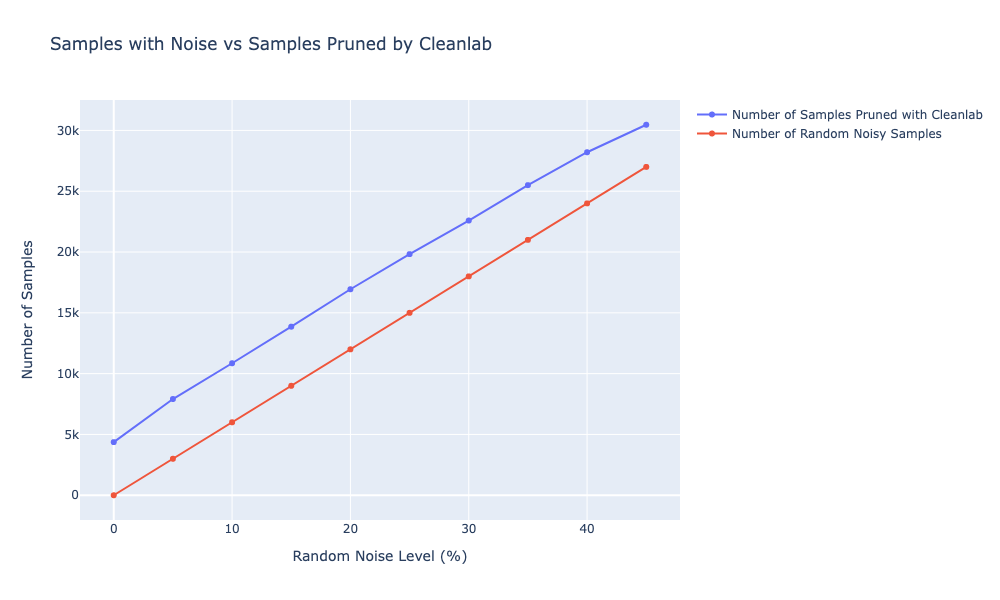I was surprised that after introducing random noise, CL would still prune the erroneous labels. The way CL algorithm works is by accurately and directly characterizing the uncertainty of label noise in the data. The foundation CL depends on is that label noise is class-conditional, depending only on the latent true class, not the data . For instance, a leopard is likely to be mistakenly labeled as a jaguar . Cleanlab takes that assumption and computes joint distribution among different classes (e.g. 3% of the data is labeled leopard (noisy label), but the true label is jaguar). The main idea is that underlying data has implications for the labeler’s decisions, and I basically took this assumption out of equation after randomly swapping labels in the dataset (e.g. I didn’t care if a certain class would be more likely to be mislabelled as another class). I would think that CL algorithm relies on this assumption heavily to guess which label to prune, but the performance was still accurate and stable. In the real-world noisy data, the mislabelling between different classes would have a stronger statistical dependence, so I can say that this example was even a bit more difficult for cleanlab.

I’ve tried to experiment with different threshold values for pruning and relabelling the images (e.g. remove 20% of the images with lowest label quality, but leave and relabel the rest). I’ve started with a threshold of 0% (e.g. relabel all labels to the ones predicted by `cleanlab` ) and then gradually increased the threshold value with a 10% step till I reached 100% prune level (e.g. remove all labels that were found to be erroneous by `cleanlab`). As before, I run these from 0 to 50% of the noise level.On the graph, I plotted the accuracy of the models trained with training sets that were fixed with different threshold values. For example, `100% Prune / 0% Relabel` indicates the accuracy of the model when all erroneous samples and their labels were deleted, while `0% Prune / 100% Relabel` shows the accuracy of the model when all of the samples were left but relabelled.Looking at the graph, I can say that `cleanlab` definitely does a great job at identifying labels, but not necessarily at fixing them automatically. As soon as I increase the % of labels that I’d like to relabel, the accuracy starts to go down in linear way. The training set with 100% of pruning got the highest accuracy, while the training set with all labels relabelled got the worst accuracy on the fixed model.As a next step, I can try to see what happens if we only remove a certain % of erroneous samples, but leave the labels of the other erroneous samples as they are. (edited)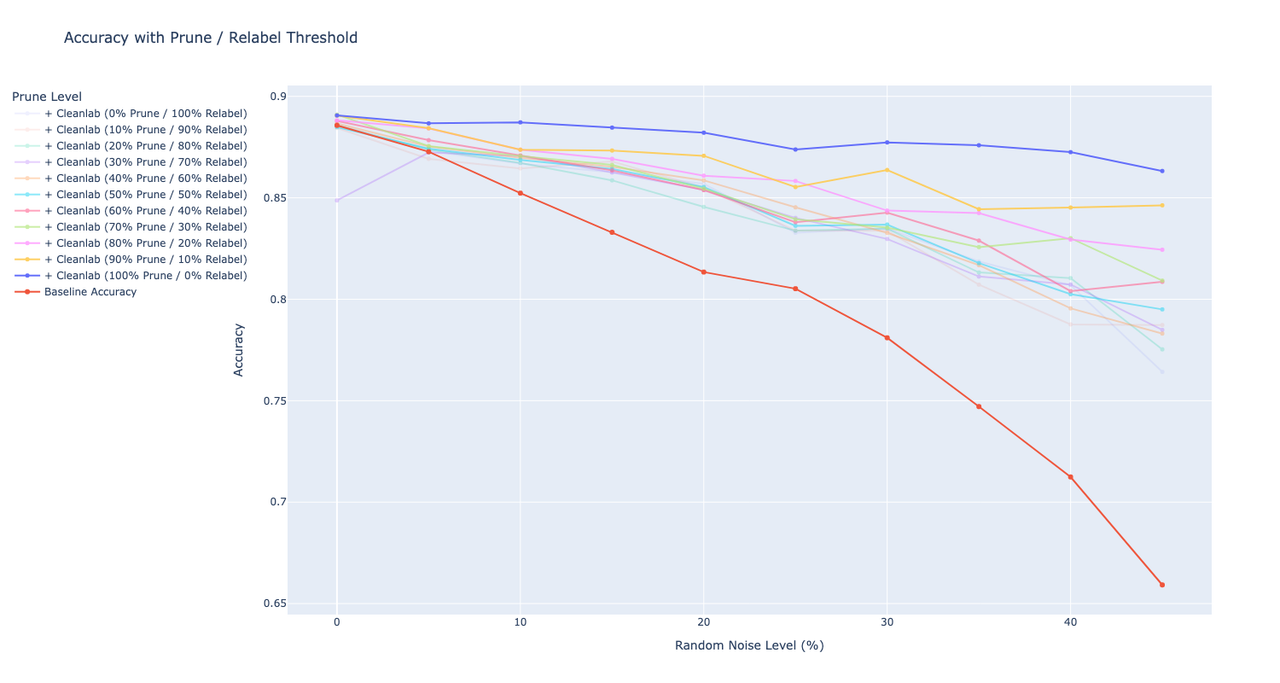I also run this pipeline on the Roman Dataset (DCAI). This dataset is quite noisy, so there’s not a ton of improvement on 0-10% of the noise level. However, as I introduce more noisy labels, looks like `cleanlab` is still able to pick them up. Running a few more trials to see what’s the performance with different Prune vs Relabel threshold.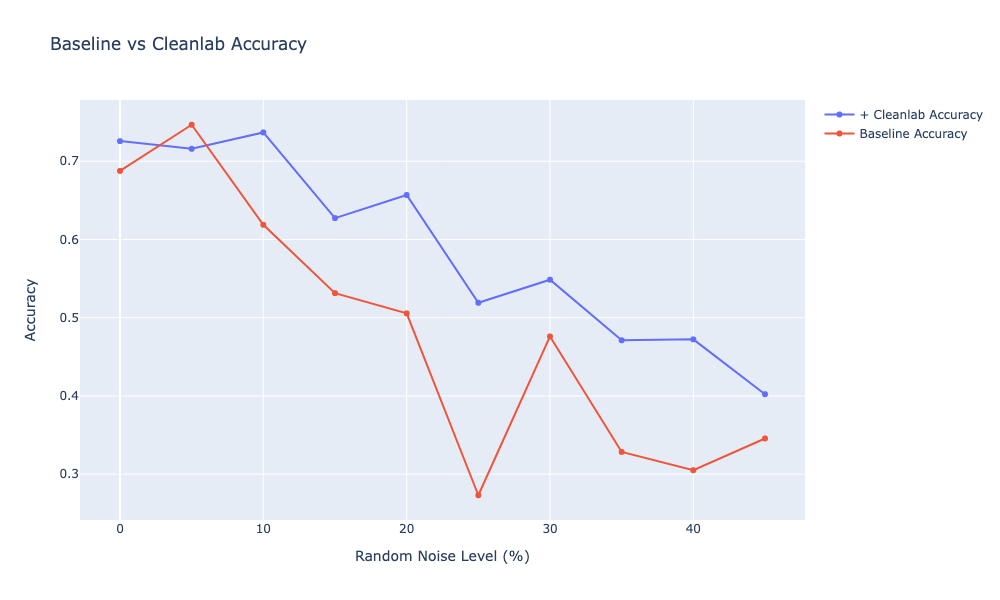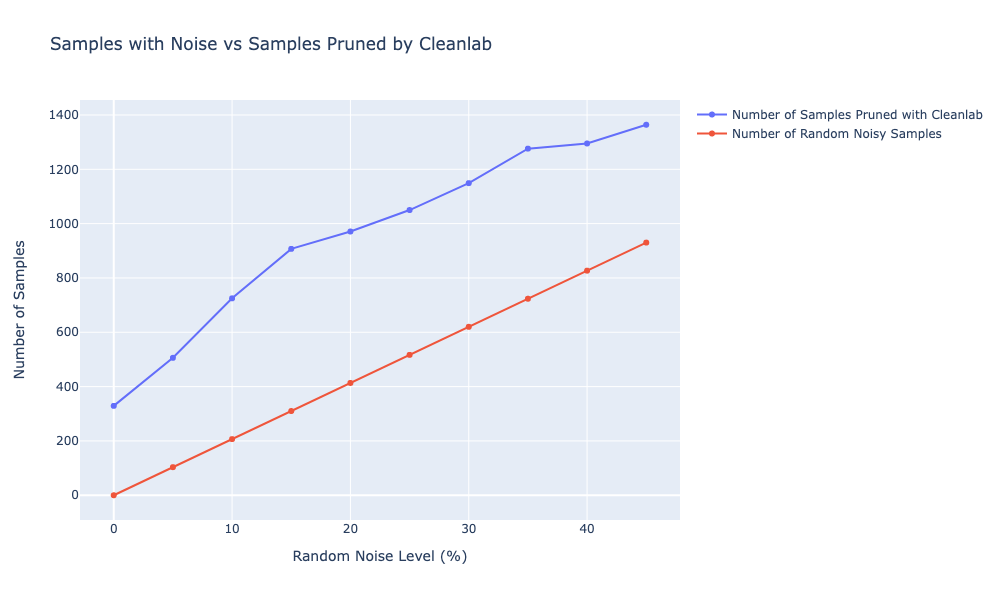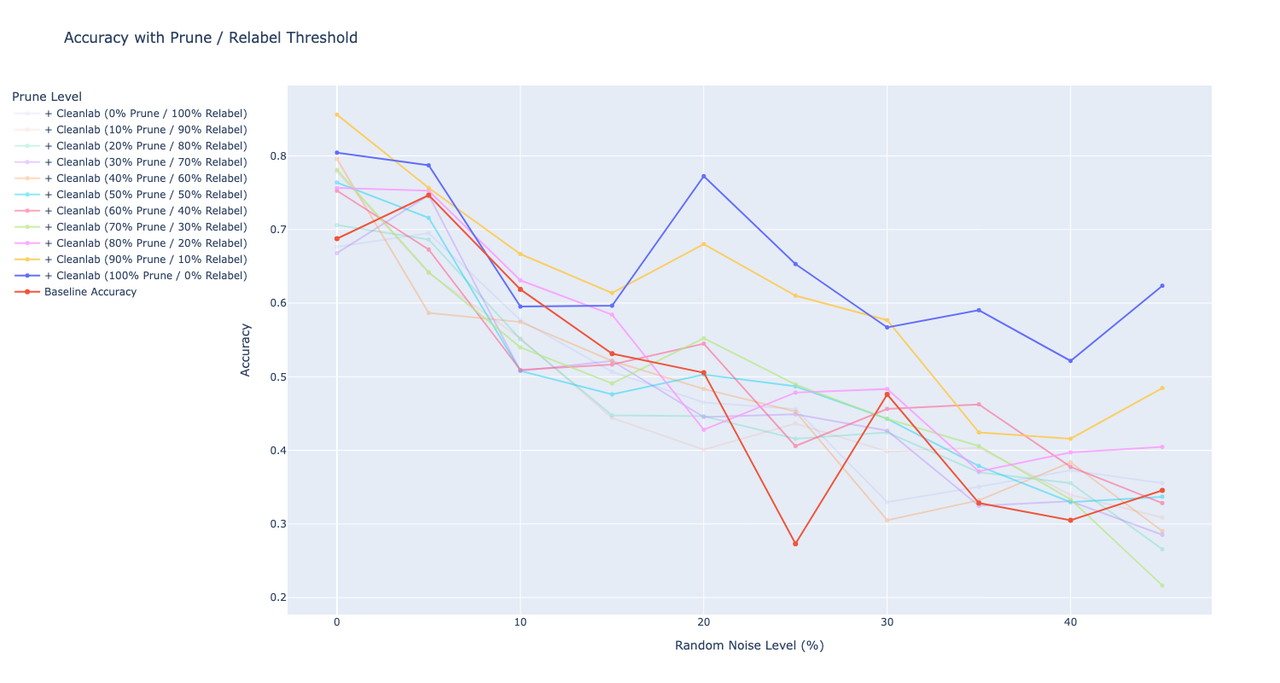View Blog Post

# GSoC Blog | Activeloop | Week 7

lowlypalace
Published: 09/11/2022

This week, I worked on running the experiments on a variety of datasets.

Here are a few exeriments that I did to benchmark `cleanlab` performance of three different datasets (MNIST Roman (DCAI), Flower 102, Fashion MNIST). For all experiments, I used a fixed model and applied resizing and normalization to the original images.

What do 1️⃣, 2️⃣, 3️⃣, 4️⃣ mean? I re-run the entire fitting with a a few random seeds to get an estimate of the variance between the accuracies of `baseline` and `cleanlab`.

1️⃣  = `seed(0)`

2️⃣  = `seed(1)`

3️⃣  = `seed(123)`

4️⃣ = `seed(42)`

## First Training Run

 Roman MNIST (DCAI) Flower 102 Fashion MNIST KMNIST Baseline Accuracy 1️⃣ 0.7945  2️⃣ 0.8511  3️⃣ 0.7699 1️⃣ 0.6568  2️⃣ 0.6176  3️⃣ 0.6274 1️⃣ 0.8958  2️⃣ 0.8944  3️⃣ 0.8987 + Cleanlab Accuracy 1️⃣ 0.7933 → -0.0012 ⬇️ 2️⃣ 0.8031 → -0.048 ⬇️ 3️⃣ 0.7109 → -0.059 ⬇️ 1️⃣ 0.5421 → -0.1147 ⬇️ 2️⃣ 0.5441 → -0.0735 ⬇️ 3️⃣ 0.5647 → -0.0627 ⬇️ 1️⃣ 0.8992 → 0.0034 ⬆️ 2️⃣ 0.8951 → 0.0007 ⬆️ 3️⃣ 0.8866 → -0.0121 ⬇️ Parameters `batch_size = 8 model = resnet18() train_shuffle = True test_shuffle = False train_split = None epochs = 10` `batch_size = 16 model = resnet18() train_shuffle = True test_shuffle = False train_split = None epochs = 10` `batch_size = 32 model = resnet18() train_shuffle = True test_shuffle = False train_split = None epochs = 10` Transform `Resize((224, 224)), ToTensor(), Normalize( [0.485, 0.456, 0.406], [0.229, 0.224, 0.225] )` `Resize((224, 224)), ToTensor(), Normalize( [0.485, 0.456, 0.406], [0.229, 0.224, 0.225] )` `ToTensor(), Normalize((0.), (1.))` `ToTensor(), Normalize((0.), (1.))` `Resize((300, 300)), ToTensor(), Normalize( [0.485, 0.456, 0.406], [0.229, 0.224, 0.225] )` Network `resnet = models.resnet18() resnet.fc = nn.Linear(resnet.fc.in_features, 10)` `resnet = models.resnet18() resnet.fc = nn.Linear(resnet.fc.in_features, 102)` `resnet = models.resnet18() resnet.conv1 = nn.Conv2d(1, 64, kernel_size=7, stride=2, padding=3, bias=False)` `resnet.fc = nn.Linear(resnet.fc.in_features, 10)` `resnet = models.resnet18() resnet.conv1 = nn.Conv2d(1, 64, kernel_size=7, stride=2, padding=3, bias=False)` `resnet.fc = nn.Linear(resnet.fc.in_features, 10)` `resnet = models.resnet18() resnet.fc = nn.Linear(resnet.fc.in_features, 47)` Parameters `batch_size = 8 model = resnet18() train_shuffle = True test_shuffle = False train_split = None` `epochs = 10` `batch_size = 16 model = resnet18() train_shuffle = True test_shuffle = False train_split = None epochs = 10` `batch_size = 32 model = resnet18() train_shuffle = True test_shuffle = False train_split = None epochs = 10` `batch_size = 64 model = resnet18() train_shuffle = True test_shuffle = False train_split = None epochs = 10` `batch_size = 32 model = resnet18() train_shuffle = True test_shuffle = False train_split = None epochs = 10` Number of Classes 10 102 10 10 47 Images Dimension 224x224 224x224 28x28 28x28 300x300

## Training with 20 Epochs

In the results below, I used `epochs = 20` instead of `epochs = 10`. The rest of the parameters (e.g. Network, Transform) are unchanged.

 MNIST Roman Flower 102 MNIST Fashion KMNIST Describable Textures Dataset Baseline Accuracy 1️⃣ 0.6875  2️⃣ 0.7736  3️⃣ 0.6617 4️⃣ 0.7945  Mean = 0.7293 1️⃣ 0.5421  2️⃣ 0.5617  3️⃣ 0.6578  4️⃣ 0.6294 1️⃣ 0.891  2️⃣ 0.8977 3️⃣ 0.8977 + Cleanlab Accuracy 1️⃣ 0.7257 → 0.0382 ⬆️ 2️⃣ 0.8400 → 0.0664 ⬆️  3️⃣ 0.8511 → 0.1894 ⬆️ 4️⃣ 0.8757 → 0.0812 ⬆️ Mean = 0.8231 Mean Difference = 0.0938 ⬆️ 1️⃣ 0.6117 → 0.0696 ⬆️ 2️⃣ 0.6254 → 0.0833 ⬆️ 3️⃣ 0.5598 → -0.098 ⬇️ 4️⃣ 0.5705 → -0.0589 ⬇️ 1️⃣ 0.8982  3️⃣ 0.897 Parameters `batch_size = 8 model = resnet18() train_shuffle = True test_shuffle = False train_split = None` `epochs = 20` `batch_size = 16 model = resnet18() train_shuffle = True test_shuffle = False train_split = None` `epochs = 20` `batch_size = 32 model = resnet18() train_shuffle = True test_shuffle = False train_split = None` `epochs = 20` `batch_size = 64 model = resnet18() train_shuffle = True test_shuffle = False train_split = None` `epochs = 20`

## Training with 30 Epochs

In the results below, I used `epochs = 30` instead of `epochs = 20`. The rest of the parameters (e.g. Network, Transform) are unchanged.

MNIST Roman Flower 102 MNIST Fashion KMNIST Describable Textures Dataset
<mark>Baseline Accuracy</mark>

0.8425

0.8831

0.8769

0.8560

Mean = 0.8646

<mark>+ Cleanlab Accuracy</mark>

0.8646 → 0.0221

0.8228 → -0.0602

0.8696 → -0.0073

0.8720 → 0.0159

Mean = 0.8573

Mean Difference = 0.0073

Parameters `batch_size = 8 model = resnet18() train_shuffle = True test_shuffle = False train_split = None` `epochs = 30` `batch_size = 16 model = resnet18() train_shuffle = True test_shuffle = False train_split = None` `epochs = 30` `batch_size = 32 model = resnet18() train_shuffle = True test_shuffle = False train_split = None` `epochs = 30` `batch_size = 64 model = resnet18() train_shuffle = True test_shuffle = False train_split = None` `epochs = 30`

## Training with Resnet50

In the results below, all of the parameters stay the same as in run above, but this time I changed the network to `resnet50()` instead of `resnet18()`. Epochs are also back to `epochs = 20`.

 MNIST Roman Flower 102 MNIST Fashion Describable Textures Dataset Baseline Accuracy 1️⃣ 0.7835  2️⃣ 0.7589  3️⃣ 0.8068  4️⃣ 0.8560 + Cleanlab Accuracy 1️⃣ 0.8044  2️⃣ 0.8560  3️⃣ 0.8376  4️⃣ 0.7859 Parameters `batch_size = 8 model = resnet50() train_shuffle = True test_shuffle = False train_split = None` `epochs = 20` `batch_size = 16 model = resnet50() train_shuffle = True test_shuffle = False train_split = None` `epochs = 20` `batch_size = 32 model = resnet50() train_shuffle = True test_shuffle = False train_split = None` `epochs = 20` `batch_size = 64 model = resnet50() train_shuffle = True test_shuffle = False train_split = None` `epochs = 20` `batch_size = 8 model = resnet50() train_shuffle = True test_shuffle = False train_split = None` `epochs = 20`

## Training with Validation Set

In the results below, all of the parameters stay the same as in run above, however, in this run I tried to run trainings with validation set. Therefore, 20% of the dataset is used for the internal training validation. In other datasets where the validation set exist, it’s used as a validation set. I set the model back to `resnet18()` as it seems it gives better baseline accuracies over three datasets.

MNIST Roman Flower 102 MNIST Fashion   Describable Textures Dataset
<mark>Baseline Accuracy</mark>

1️⃣ 0.6432

2️⃣ 0.6445

3️⃣ 0.6777

4️⃣ 0.6383

Mean = 0.6509

0.6421

0.6578

0.6823

0.6372

Mean = 0.6549

0.8974

0.891

0.8906

0.894

Mean = 0.89325

0.9425

0.9439

0.9436

0.9364

Mean = 0.9416

<mark>+ Cleanlab Accuracy</mark>

1️⃣ 0.5584

2️⃣ 0.5879

3️⃣ 0.6076

4️⃣ 0.4587 Mean = 0.5531

Parameters `batch_size = 8 model = resnet18() train_shuffle = True test_shuffle = False` `train_split=ValidSplit(cv=5, stratified=False) valid_shuffle = False` `epochs = 20` `batch_size = 16 model = resnet18() train_shuffle = True test_shuffle = False` `train_split=predefined_split(Dataset(valid_data, valid_labels)) valid_shuffle = False` `epochs = 20` `batch_size = 32 model = resnet18() train_shuffle = True test_shuffle = False` `train_split=ValidSplit(cv=5, stratified=False) valid_shuffle = False` `epochs = 20` `batch_size = 64 model = resnet18() train_shuffle = True test_shuffle = False` `train_split=ValidSplit(cv=5, stratified=False)` `valid_shuffle = False` `epochs = 20`

## Training with Validation Set (Stratified Sampling)

Using arbitrary random seed can result in large differences between the training and validation set distributions. These differences can have unintended downstream consequences in the modeling process. As an example, the proportion of digit X can much higher in the training set than in the validation set. To overcome this, I’m using stratified sampling (sampling from each class with equal probability) to create the validation set for the datasets where it’s not available by default (e.g. MNIST Roman, MNIST Fashion, KMNIST).

 MNIST Roman Flower 102 MNIST Fashion Describable Textures Dataset Baseline Accuracy 0.7515 0.6998 0.7958 0.8610 Mean = 0.7770 N/A 0.8928 0.8948 0.8969 0.895 Mean = 0.894875 N/A + Cleanlab Accuracy 0.6027 → -0.1488 0.8228 → 0.1230 0.8130 → 0.0172 0.6900 → -0.1709 Mean = 0.7321 Mean Difference = -0.0448 Parameters `batch_size = 8 model = resnet18() train_shuffle = True test_shuffle = False` `train_split=ValidSplit(cv=5, stratified=True) valid_shuffle = False` `epochs = 20` `batch_size = 32 model = resnet18() train_shuffle = True test_shuffle = False` `train_split=ValidSplit(cv=5, stratified=True) valid_shuffle = False` `epochs = 20` `batch_size = 64 model = resnet18() train_shuffle = True test_shuffle = False` `train_split=ValidSplit(cv=5, stratified=True)` `valid_shuffle = False` `epochs = 20`

## Training with Early Stopping

 MNIST Roman Flower 102 MNIST Fashion Describable Textures Dataset Baseline Accuracy 0.8327 0.8388 0.7921 0.8597 Mean = 0.8308 0.6705 0.6578 0.6558 0.6313 Mean = 0.6539 0.8856, 0.8916, 0.8856, 0.8917 Mean = 0.88862 + Cleanlab Accuracy 0.8683 0.8339 0.8597 0.8105 Mean = 0.8431 0.6539 0.6176 0.887 0.8904 Parameters `callbacks=[EarlyStopping(monitor='train_loss', patience=5)]` `callbacks=[EarlyStopping(monitor='train_loss', patience=5)]` `train_split=predefined_split(Dataset(valid_data, valid_labels))`

Notebooks to reproduce results:

View Blog Post

# GSoC Blog | Activeloop | Week 6

lowlypalace
Published: 09/11/2022

## What did you do this week?

This week, I've set up a pipeline for running experiments on a variety of datasets to compare the accuracies of baseline (the model trained on dirty data) and cleanlab (the model trained on clean data).

## What is coming up next?

As a next step, I will be running experiments on a variety of datasets to benchmark the accuracy.

## Did you get stuck anywhere?

Not really, it was a bit tricky to set up a pipeline in a way that's reproducible. I managed to overcome this by fixing the seeds.

View Blog Post

# GSoC Blog | Activeloop | Week 5

lowlypalace
Published: 07/19/2022

This week, I've been focusing on the high-level overview of data-centric strategies. I used Roman MNIST Dataset that was provided in the Data-Centric AI Competition. For all experiments, I used fixed Resnet50 and applied resizing and normalization to the original dataset. I also used a fixed seed to be able to replicate the runs. Here are some of the metrics I’ve been getting:

1. Fixing Labels with Cleanlab.
• Baseline Accuracy: 0.7318
• `CleanLearning` Accuracy: 0.8290
1. Automatic Augmentations with AutoAlbument (uses Faster AutoAugment algorithm)
• Baseline Accuracy: 0.7318
• `AutoAlbument` Augmentations: 0.7404
1. Augmentations with Basic Augmentations and Pre-trained Torch Policies
• Baseline Accuracy: 0.7318
• Basic Baseline Augmentations: 0.8696
• ImageNet Pre-Trained Policy: 0.8560

Here are my findings for these strategies:

• Fixing Labels with Cleanlab.
• I’m now trying out smaller `k` values for cross-validation to see to which extent it impacts the accuracy and improves the training speed. I will also try out more epoch ranges to see if this has impact on the accuracy. I’ve noticed that `cleanlab` performs well only when we already have a robust baseline model. For this specific dataset, I’ve noticed that if the accuracy of the baseline model is less than 0.7, then `cleanlab` actually has negative effect on the accuracy. I believe this is because of the confident learning algorithm, as it needs to get as accurate confidence scores for each label as possible.
• For now, the labels fixing and augmentations are applied separately. I think it would be also interesting to see how the accuracy changes after applying `CleanLearning` and then `AutoAlbument`. But I haven’t found out an easy way to get the corrected labels from CleanLearning to overwrite the initial labels of the dataset. I’ve messaged the `cleanlab` team to get their help on this.
• Automatic Augmentations with AutoAlbument.
• I’ve only used `epochs = 15` to find optimal augmentation policy. I’ll try out more epochs ranges to see if this can improve the accuracy.
• I have also used the whole train and validation datasets for finding the optimal augmentation policy. I then applied augmentations only to train dataset and validated it on the test dataset.
• Augmentations with Basic Augmentations and Pre-trained Torch Policies
• I was surprised by the accuracy improvement after applying basic transformations, such as `HorizontalFlip()``RandomCrop()`, and `RandomErasing().`
• I only applied augmentations to the train dataset.
View Blog Post

# GSoC Blog | Activeloop | Week 4

lowlypalace
Published: 07/18/2022

## What did you do this week?

This week, I've been focusing on implementing custom cross-validation algorithm to compute out-of-sample probabilities for Hub Datasets.

## What is coming up next?

As a next step, I will be working on a generic high-level pipeline that consists of fixing labels on a particular dataset, applying augmentations to a dataset and finding slices that underperform on a particular dataset.

## Did you get stuck anywhere?

Not particularly, I had a few issues with ensuring that my experiments are deterministic with PyTorch but at the end I was able to fix it.

View Blog Post